Question
Using java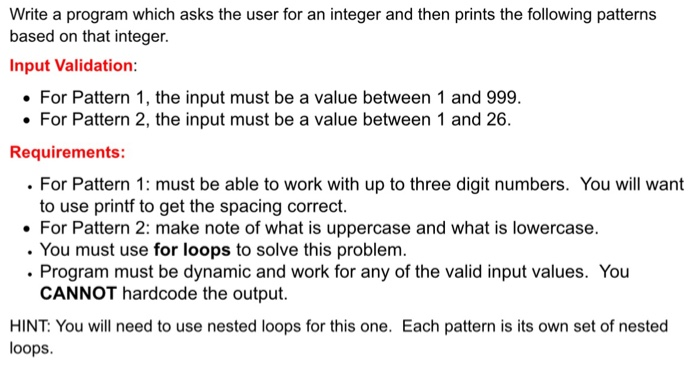Sample Outputs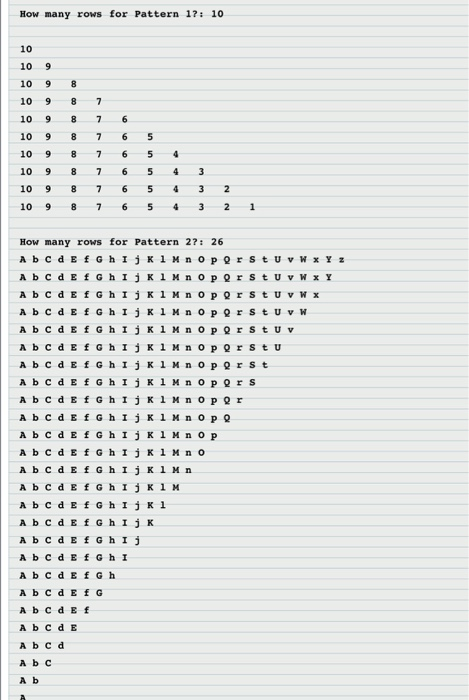import java.io.*;

import java.util.*;

class pattern

{

public static void main(String args[])

{

int p1,p2;//for pattern1 and pattern 2

int m,n;

Scanner s=new Scanner(System.in);

int flag1=1,k;

System.out.print("how many rows for pattern1 ? : ");

p1=s.nextInt();

if(p1>0 && p1<1000) flag1=0;

while(flag1==1)

{

System.out.print("how many rows for pattern1 ? : ");

p1=s.nextInt();

if(p1>0 && p1<1000) flag1=0;

}

m=p1;

System.out.println();

for(int i=1;i<=m;i++)

{

k=m;

for(int j=1;j<=i;j++)

{

System.out.print(k-- +" ");

}

System.out.println();

}

int flag2=1;

System.out.print("how many rows for pattern2 ? : ");

p2=s.nextInt();

if(p2>0 && p2<27) flag2=0;

while(flag2==1)

{

System.out.print("how many rows for pattern2 ? : ");

p2=s.nextInt();

if(p2>0 && p2<27) flag2=0;

}

n=p2;

System.out.println();

for(int i=n;i>=1;i--)

{

for(int j=1;j<=i;j++)

{

if(j%2==0)

{

System.out.print((char)(j+64+32) +" ");

}

else

{

System.out.print((char)(j+64) +" ");

}

}

System.out.println();

}

}

}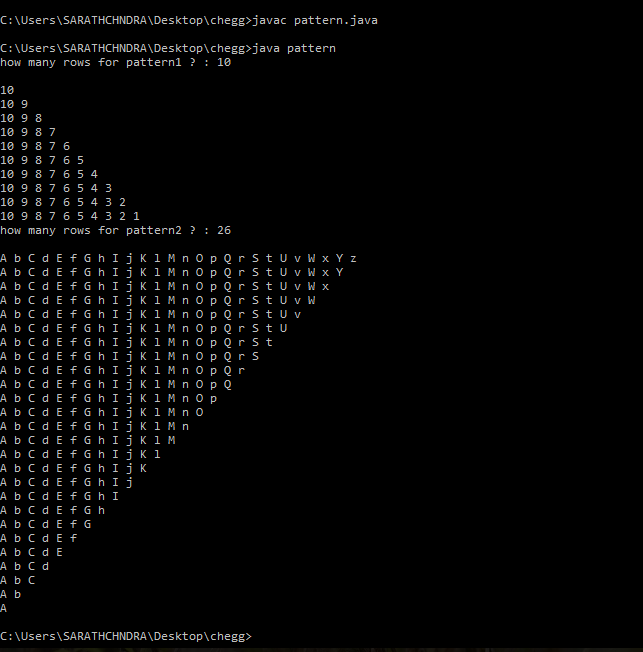#### Earn Coins

Coins can be redeemed for fabulous gifts.

Similar Homework Help Questions
• ### Exercise 1: Write a C++ program that asks the user to input an integer n followed...

Exercise 1: Write a C++ program that asks the user to input an integer n followed by n other characters that will be stored in an array. We suppose here that the user will input only lowercase characters between ‘a’ and ‘z’. We also suppose that the user will input different characters. The program will then sort these characters according to an increasing order of their alphabetical order. In this exercise, feel free to use selection sort, insertion sort, or...

• ### ANSWER IN C PLEASE (: ew History Bookmarks Window Help 13.16. Loops E ry> ECE 270...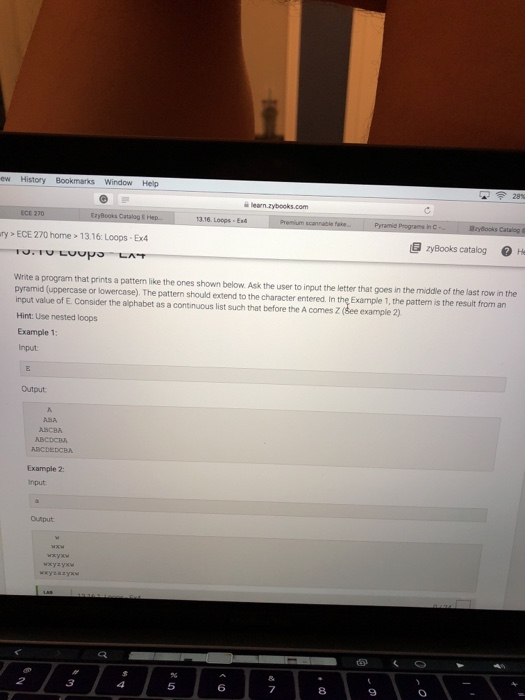ANSWER IN C PLEASE (: ew History Bookmarks Window Help 13.16. Loops E ry> ECE 270 home > 13.16 Loops-Ex4 zyBooks catalog TO.TU LOOo Write a program that prints a pattern like the ones shown below Ask the user to input the letter that goes in the middle of the last row in the pyramid (uppercase or lowercase). The pattern should extend to the character entered. In the Example 1, the pattern is the result from an input value of...

• ### Using LC3 Editor. This program accepts an integer typed in by the user, verifies that the...

Using LC3 Editor. This program accepts an integer typed in by the user, verifies that the number is valid, and if it is valid prints the binary version of the number to the display as on the following page. Call the program part2.asm. As you can see the program rejects any input which doesn't start with a + or - sign and prints "The input is invalid." to the display. Also any integer values less than -511 or greater than...

• ### C++ coding answer 5. Write a program that asks the user for a positive integer value....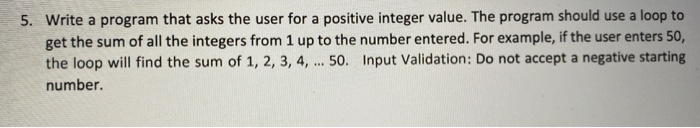C++ coding answer 5. Write a program that asks the user for a positive integer value. The program should use a loop to get the sum of all the integers from 1 up to the number entered. For example, if the user enters 50, the loop will find the sum of 1, 2, 3, 4, ... 50. Input Validation: Do not accept a negative starting number.

• ### Write a program that prints the following pattern to the screen. (no blank lines between lines)...

Write a program that prints the following pattern to the screen. (no blank lines between lines) You must use nested for loops. 1 212 32123 4321234 543212345 c++

• ### In Python: Write a well-documented (commented) program that asks the user for a 9-digit integer, computes...

In Python: Write a well-documented (commented) program that asks the user for a 9-digit integer, computes its checksum, and then prints the corresponding ISBN number. The International Standard Book Number (ISBN) is a 10-digit code that uniquely specifies a book. The rightmost digit is a checksum digit that can be uniquely determined from the other 9 digits, from the condition that d1 + 2d2 +3d3 + ... + 10d10 must be a multiple of 11 (here di denotes the ith...

• ### Design a Java program that asks the user to enter an integer number n and then...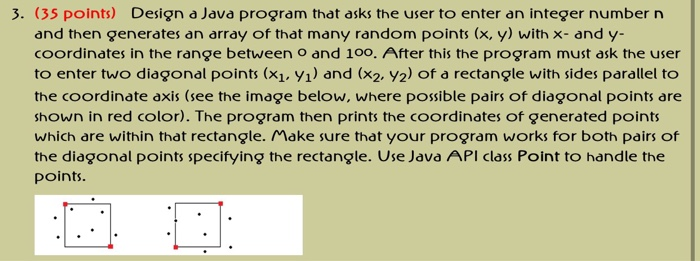Design a Java program that asks the user to enter an integer number n and then generates an array of that many random points (x, y) with x- and y-coordinates in the range between 0 and 100. After this the program must ask the user to enter two diagonal points (x_1, y_1) and (x_2, y_2) of a rectangle with sides parallel to the coordinate axis (see the image below, where possible pairs of diagonal points are shown in red color)....

• ### Write a Java program which asks the user to enter an integer x. This program prints...

Write a Java program which asks the user to enter an integer x. This program prints the remainder of x when it is divided by 3. Example Pl ea s e e n t e r an i n t e g e r : 3 remainde r=0 Pl ea s e e n t e r an i n t e g e r : 17 remainde r=2

• ### C++ Write a complete program that will prompt the user for a digit in the range...

C++ Write a complete program that will prompt the user for a digit in the range 5 to 15. Using that digit to determin scale display the graphic shown below. Your program must use a nested loop. You may not use the string class SAMPLE RUN: Input an integer in the range 5 to 20: 100 Incorrect. The number is out of range. Input an integer in the range 5 to 20: 10 * ** *** **** ***** ****** *******...

• ### Write a python program that does the following. a. It asks the user to enter a...

Write a python program that does the following. a. It asks the user to enter a 5-digit integer value, n. b. The program reads a value entered by the user. If the value is not in the right range, the program should terminate. c. The program calculates and stores the 5 individual digits of n. d. The program outputs a “bar code” made of 5 lines of stars that represent the digits of the number n. For example, the following...